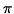Optimization problems

1. Find the rectangle which has the maximum area among the rectangles whose perimeter is 4 m.The rectangle with the maximum area is the square of side 1 m

2. Find the dimensions so that the cost of building a cuboid container of 16 m3 volume is minimum, knowing that its height must be 2 m, and the costs are: 30€/m2 for the base, 36€/m2 for the lateral sides and 42€/m2 for the cover.The dimensions must be 2m x 2√2m x 2√2 m

Exercises:
1) Find two nonnegative numbers whose sum is 9 and so that the product of one number and the square of the other number is a maximum.

2) A container in the shape of a right circular cylinder with no top has surface area 3m2. What height h and base radius r will maximize the volume of the cylinder ?

Solutions: 1) 3 and 6; 2) r = h = 1 m